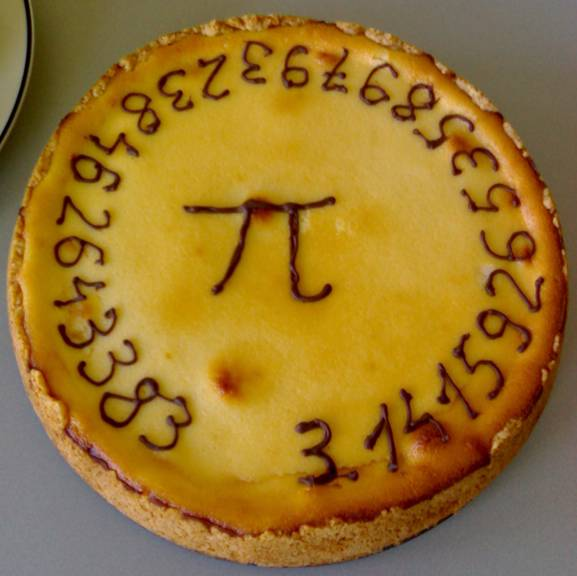# It is Pi DayHey, Happy Rounded up Pi day everybody – (3.14159 can be rounded up to 3.1416)

## What the heck is Pi ?

Pi (Greek letter “π”) is the symbol used in mathematics to represent a constant — the ratio of the circumference of a circle to its diameter — which is approximately 3.14159265, and what is “magical” about it is that it works for all circles of any size, Pi remains the same.

OK, so what can I use it for?

Well how about the observation that it makes geometry problems involving circles very easy to resolve, for example …

The area of a circle.

A = πr2

Where ‘r’ is the radius (distance from the center to the edge of the circle). Incidentally, this is the origin of the joke “Pies aren’t square, they’re round!”

Now let’s build on that and go for the volume of a cylinder.

V = πr2h

Basically take the above area of a circle and simply multiply the answer by the height of the cylinder.

Now that is indeed simple.

There is much more you can do with it of course, the above is just a quick example of how using this rather strange irrational constant number that can only be expressed by giving it a symbol of its very own, yields solutions to problems that would otherwise be quite challenging.

Did you also know that it gets used, not just in geometry, but also in cosmologynumber theorystatisticsfractalsthermodynamicsmechanics and electromagnetism – and so that is what makes this mathematical constant truly special.

Bottom line: if you need to calculate something that involves circles, ellipses or spheres, then this is an extremely valuable tool to deploy.

## Hat Tip to the Birthday boy Einstein

Einstein had the wisdom to be born on Pi day, 14th March … now that was indeed a smart move on his part :-)

OK, on to the celebrations …

## Music constructed using π

1.